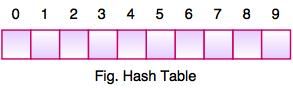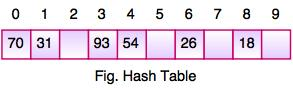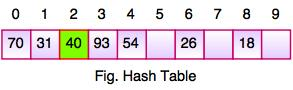# Hashing in Data Structure

## What is Hashing?

• Hashing is the process of mapping large amount of data item to smaller table with the help of hashing function.
• Hashing is also known as Hashing Algorithm or Message Digest Function.
• It is a technique to convert a range of key values into a range of indexes of an array.
• It is used to facilitate the next level searching method when compared with the linear or binary search.
• Hashing allows to update and retrieve any data entry in a constant time O(1).
• Constant time O(1) means the operation does not depend on the size of the data.
• Hashing is used with a database to enable items to be retrieved more quickly.
• It is used in the encryption and decryption of digital signatures.

## What is Hash Function?

• A fixed process converts a key to a hash key is known as a Hash Function.
• This function takes a key and maps it to a value of a certain length which is called a Hash value or Hash.
• Hash value represents the original string of characters, but it is normally smaller than the original.
• It transfers the digital signature and then both hash value and signature are sent to the receiver. Receiver uses the same hash function to generate the hash value and then compares it to that received with the message.
• If the hash values are same, the message is transmitted without errors.

## What is Hash Table?

• Hash table or hash map is a data structure used to store key-value pairs.
• It is a collection of items stored to make it easy to find them later.
• It uses a hash function to compute an index into an array of buckets or slots from which the desired value can be found.
• It is an array of list where each list is known as bucket.
• It contains value based on the key.
• Hash table is used to implement the map interface and extends Dictionary class.
• Hash table is synchronized and contains only unique elements.

•• The above figure shows the hash table with the size of n = 10. Each position of the hash table is called as Slot. In the above hash table, there are n slots in the table, names = {0, 1, 2, 3, 4, 5, 6, 7, 8, 9}. Slot 0, slot 1, slot 2 and so on. Hash table contains no items, so every slot is empty.
• As we know the mapping between an item and the slot where item belongs in the hash table is called the hash function. The hash function takes any item in the collection and returns an integer in the range of slot names between 0 to n-1.
• Suppose we have integer items {26, 70, 18, 31, 54, 93}. One common method of determining a hash key is the division method of hashing and the formula is :

• Hash Key = Key Value % Number of Slots in the Table

• Division method or reminder method takes an item and divides it by the table size and returns the remainder as its hash value.

• Data ItemValue % No. of SlotsHash Value
2626 % 10 = 66
7070 % 10 = 00
1818 % 10 = 88
3131 % 10 = 11
5454 % 10 = 44
9393 % 10 = 33• After computing the hash values, we can insert each item into the hash table at the designated position as shown in the above figure. In the hash table, 6 of the 10 slots are occupied, it is referred to as the load factor and denoted by, λ = No. of items / table size. For example , λ = 6/10.
• It is easy to search for an item using hash function where it computes the slot name for the item and then checks the hash table to see if it is present.
• Constant amount of time O(1) is required to compute the hash value and index of the hash table at that location.

## Linear Probing

• Take the above example, if we insert next item 40 in our collection, it would have a hash value of 0 (40 % 10 = 0). But 70 also had a hash value of 0, it becomes a problem. This problem is called as Collision or Clash. Collision creates a problem for hashing technique.
• Linear probing is used for resolving the collisions in hash table, data structures for maintaining a collection of key-value pairs.
• Linear probing was invented by Gene Amdahl, Elaine M. McGraw and Arthur Samuel in 1954 and analyzed by Donald Knuth in 1963.
• It is a component of open addressing scheme for using a hash table to solve the dictionary problem.
• The simplest method is called Linear Probing. Formula to compute linear probing is:

• P = (1 + P) % (MOD) Table_size
For example,If we insert next item 40 in our collection, it would have a hash value of 0 (40 % 10 = 0). But 70 also had a hash value of 0, it becomes a problem.

Linear probing solves this problem:

P = H(40)
44 % 10 = 0
Position 0 is occupied by 70. so we look elsewhere for a position to store 40.

Using Linear Probing:
P= (P + 1) % table-size
0 + 1 % 10 = 1
But, position 1 is occupied by 31, so we look elsewhere for a position to store 40.

Using linear probing, we try next position : 1 + 1 % 10 = 2
Position 2 is empty, so 40 is inserted there.